• 二进制是计算技术中广泛采用的一种数制。二进制数据是用0和1两个数码...二进制转换十进制公式：abcd.efg(2)=d*2^0+c*2^1+b*2^2+a*2^3+e*2^-1+f*2^-2+g*2^-3(10)例如二进制数据110.11，其权的大小顺序为2^2、2^1、2^0...

二进制是计算技术中广泛采用的一种数制。二进制数据是用0和1两个数码来表示的数。它的基数为2，进位规则是“逢二进一”，借位规则是“借一当二”。二进制数据也是采用位置计数法，其位权是以2为底的幂。

二进制转换十进制公式：

abcd.efg(2)=d*2^0+c*2^1+b*2^2+a*2^3+e*2^-1+f*2^-2+g*2^-3(10)

例如二进制数据110.11，其权的大小顺序为2^2、2^1、2^0、2^-1、2^-2。对于有n位整数，m位小数的二进制数据用加权系数展开式表示，可写为：

(a(n-1)a(n-2)…a(-m))2＝a(n-1)×2^(n-1)+a(n-2)×2^(n-2)+……+a(1)×2^1+a(0)×2^0+a(-1)×2^(-1)+a(-2)×2^(-2)+……+a(-m)×2^(-m)

二进制数据一般可写为：(a(n-1)a(n-2)…a(1)a(0).a(-1)a(-2)…a(-m))2。

注意：

1、式中aj表示第j位的系数，它为0和1中的某一个数。

2、a(n-1)中的(n-1)为下标，输入法无法打出所以用括号括住，避免混淆。

3、2^2表示2的平方，以此类推。

二进制转为十进制计算方法举例

二进制转为十进制的时候，先把二进制从高位(最左边的“1”)开始按从上到下的顺序写出 ，第一位就是最后的商 “2÷2 = 1 余0 “，余数肯定是加零。其他位数如果有”1“(原来的余数)，就先乘以”2“再加”1“。

下面就是从第一位开始乘以2加余数的方法算回去

例如 100101110

1…………0x2+1=1…………余数为1

0…………1x2+0=2………… 余数为0

0 …………2x2+0=4 ………… 余数为0

1 …………4x2+1=9……………… 余数为1

0…………9x2+0=18 ……………… 余数为0

1 …………18x2+1=37 …………余数为1

1…………… 37x2+1=75…………余数为1

1………………75x2+1=151………… 余数为1

0………………151x2+0=302 ………… 余0

所以得到十进制数302

另：1*2^8+0*2^7+0*2^6+1*2^5+0*2^4+1*2^3+1*2^2+1*2^1+0*2^0=302

展开全文• 目录十进制转换为任意进制任意进制转换为十进制 十进制转换为任意进制 //将10进制数num转换为d进制数 void convert_base(int num, int d) { int k; stack<int> stk; while (num) { k = num % d; num = ...

十进制转换为任意进制

//将10进制数num转换为d进制数
void convert_base(int num, int d)
{
int k;
stack<int> stk;
while (num)
{
k = num % d;
num = num / d;
stk.push(k);
}

while (!stk.empty())
{
k = stk.top();
stk.pop();
printf("%x", k);
}
}

任意进制转换为十进制

#define MAXN 64     //64位CPU
//将s的d进制数转化为10进制数
int restore(const char s[MAXN], int d)
{
int res = 0;
int k;

for (int i = 0; s[i] != '\0'; i++)
{
if (s[i] >= '0' && s[i] <= '9') k = s[i] - '0';
else if (s[i] >= 'A' && s[i] <= 'Z') k = s[i] - 'A' + 10;
else k = s[i] - 'a' + 10;

res = res * d + k;
}
return res;
}

展开全文算法 编程基础 进制转换
• c语言二进制转换成十进制方法发布时间：2020-04-09 09:52:38来源：亿速云阅读：648作者：小新今天小编给大家分享的是c语言二进制转换成十进制方法，很多人都不太了解，今天小编为了让大家更加了解二进制转换成...

c语言二进制转换成十进制的方法

发布时间：2020-04-09 09:52:38

来源：亿速云

阅读：648

作者：小新

今天小编给大家分享的是c语言二进制转换成十进制的方法，很多人都不太了解，今天小编为了让大家更加了解二进制转换成十进制的方法，所以给大家总结了以下内容，一起往下看吧。一定会有所收获的哦。c语言二进制怎么转换十进制？

按照如下步骤即可用C语言把二进制数转换成十进制数的程序：

1、首先在主函数中设置成函数Sum，另外定义了一个数组array，用于存放输入的八位二进制数。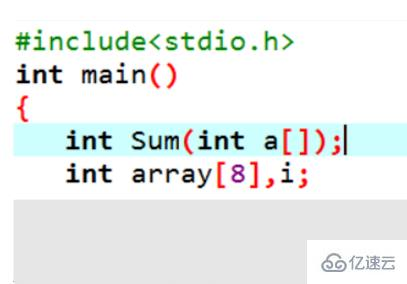2、然后使用了一个for循环语句，用于输入八位二进制数。在scanf函数里，在%d之间加一个1，然后使用printf函数输出，并且调用Sum函数，数组名作为实参。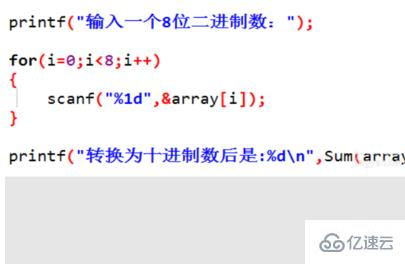3、在Sum函数中，声明了一个power函数，在这里n为指数，term为每一位数转换为十进制后的的数，如1*(2*2)，sum为总和。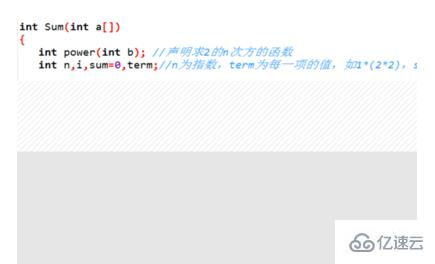4、然后使用一个for循环语句，i用于控制循环的次数，n从最高的指数7开始每次循环减一，如果指数大于或等于零的，就进行循环，调用power函数时，将n作为实参，最后将和返回。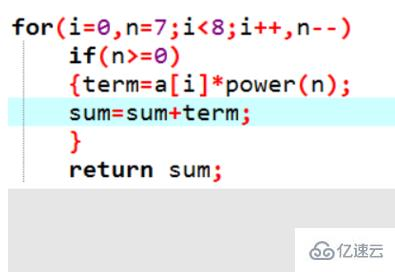5、在power函数中，如果b等于零，即指数为零，则返回1，否则就进入一个循环，j用于控制循环次数。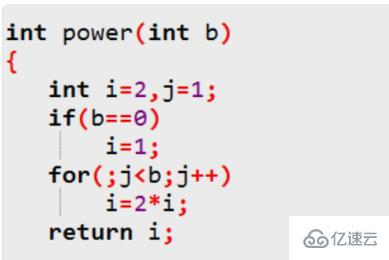6、最后看一下运行结果，这个C语言程序既可以把二进制数成功转换成十进制数了。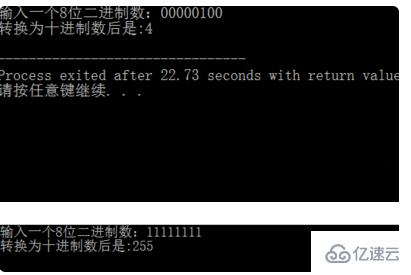以上就是c语言二进制转换成十进制方法的简略介绍，当然详细使用上面的不同还得要大家自己使用过才领会。如果想了解更多，欢迎关注亿速云行业资讯频道哦！

展开全文• 中文名十进制转二进制外文名Decimal system to binary system适用领域电子、编程、编码应用学科数学十进制转二进制二进制转十进制编辑语音十进制转二进制方法一小数点前或者整数要从右到左用二进制的每个数去乘以2的...

十进制数转换为二进制数时，由于整数和小数的转换方法不同，所以先将十进制数的整数部分和小数部分分别转换后，再加以合并。

中文名

十进制转二进制

外文名

Decimal system to binary system适用领域

电子、编程、编码

应用学科

数学

十进制转二进制二进制转十进制

编辑

语音

十进制转二进制方法一

小数点前或者整数要从右到左用二进制的每个数去乘以2的相应次方并递增,小数点后则是从左往右乘以二的相应负次方并递减。

例如：二进制数1101.01转化成十进制

1101.01(2)=1*20+0*21+1*22+1*23+0*2-1+1*2-2=1+0+4+8+0+0.25=13.25(10)

所以总结起来通用公式为：

abcd.efg(2)=d*20+c*21+b*22+a*23+e*2-1+f*2-2+g*2-3(10)

十进制转二进制方法二

或者用下面这种方法：

把二进制数首先写成加权系数展开式，然后按十进制加法规则求和。这种做法称为"按权相加"法。

2的0次方是1(任何数的0次方都是1，0的0次方无意义)

2的1次方是2

2的2次方是4

2的3次方是8

2的4次方是16

2的5次方是32

2的6次方是64

2的7次方是128

2的8次方是256

2的9次方是512

2的10次方是1024

2的11次方是2048

2的12次方是4096

2的13次方是8192

2的14次方是16384

2的15次方是32768

2的16次方是65536

2的17次方是131072

2的18次方是262144

2的19次方是524288

2的20次方是1048576

即：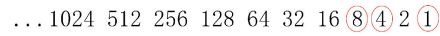2的次方

此时，1101=8+4+0+1=13

再比如：二进制数100011转成十进制数可以看作这样：

数字中共有三个1 即第六位一个，第二位一个，第一位一个(从右到左)，然后对应十进制数即2的0次方+2的1次方+2的5次方， 即

100011=32+0+0+0+2+1=35

十进制转二进制十进制转二进制

编辑

语音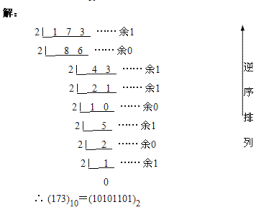1. 十进制整数转换为二进制整数十进制整数转换为二进制整数采用"除2取余，逆序排列"法。具体做法是：用2整除十进制整数，可以得到一个商和余数；再用2去除商，又会得到一个商和余数，如此进行，直到商为小于1时为止，然后把先得到的余数作为二进制数的低位有效位，后得到的余数作为二进制数的高位有效位，依次排列起来。

十进制整数转二进制

如：255=(11111111)B

255/2=127=====余1

127/2=63======余1

63/2=31=======余1

31/2=15=======余1

15/2=7========余1

7/2=3=========余1

3/2=1=========余1

1/2=0=========余1

789=1100010101(B)

789/2=394 余1 第10位

394/2=197 余0 第9位

197/2=98 余1 第8位

98/2=49 余0 第7位

49/2=24 余1 第6位

24/2=12 余0 第5位

12/2=6 余0 第4位

6/2=3 余0 第3位

3/2=1 余1 第2位

1/2=0 余1 第1位

原理：

众所周知，二进制的基数为2，我们十进制化二进制时所除的2就是它的基数。谈到它的原理，就不得不说说关于位权的概念。某进制计数制中各位数字符号所表示的数值表示该数字符号值乘以一个与数字符号有关的常数，该常数称为 “位权 ” 。位权的大小是以基数为底，数字符号所处的位置的序号为指数的整数次幂。十进制数的百位、十位、个位、十分位的权分别是10的2次方、10的1次方、10的0次方，10的-1次方。二进制数就是2的n次幂。

按权展开求和正是非十进制化十进制的方法。

下面我们开讲原理，举个十进制整数转换为二进制整数的例子，假设十进制整数A化得的二进制数为edcba 的形式，那么用上面的方法按权展开， 得

A=a(2^0)+b(2^1)+c(2^2)+d(2^3)+e(2^4) (后面的和不正是化十进制的过程吗)

假设该数未转化为二进制,除以基数2得

A/2=a(2^0)/2+b(2^1)/2+c(2^2)/2+d(2^3)/2+e(2^4)/2

注意：a除不开二，余下了！其他的绝对能除开，因为他们都包含2，而a乘的是1，他本身绝对不包含因数2，只能余下。

商得：

b(2^0)+c(2^1)+d(2^2)+e(2^3)，再除以基数2余下了b，以此类推。

当这个数不能再被2除时，先余掉的a位数在原数低，而后来的余数数位高，所以要把所有的余数反过来写。正好是edcba

2．十进制小数转换为二进制小数

十进制小数转换成二进制小数采用"乘2取整，顺序排列"法。具体做法是：用2乘十进制小数，可以得到积，将积的整数部分取出，再用2乘余下的小数部分，又得到一个积，再将积的整数部分取出，如此进行，直到积中的小数部分为零，此时0或1为二进制的最后一位。或者达到所要求的精度为止。

然后把取出的整数部分按顺序排列起来，先取的整数作为二进制小数的高位有效位，后取的整数作为低位有效位。

十进制小数转二进制

如：0.625=(0.101)B

0.625*2=1.25======取出整数部分1

0.25*2=0.5========取出整数部分0

0.5*2=1==========取出整数部分1

再如：0.7=(0.1 0110 0110...)B

0.7*2=1.4========取出整数部分1

0.4*2=0.8========取出整数部分0

0.8*2=1.6========取出整数部分1

0.6*2=1.2========取出整数部分1

0.2*2=0.4========取出整数部分0

0.4*2=0.8========取出整数部分0

0.8*2=1.6========取出整数部分1

0.6*2=1.2========取出整数部分1

0.2*2=0.4========取出整数部分0

原理：

关于十进制小数转换为二进制小数

假设一十进制小数B化为了二进制小数0.ab的形式，同样按权展开，得

B=a(2^-1)+b(2^-2)

因为小数部分的位权是负次幂，所以我们只能乘2，得

2B=a+b(2^-1)

注意a变成了整数部分，我们取整数正好是取到了a，剩下的小数部分也如此。

值得一提的是，小数部分的按权展开的数位顺数正好和整数部分相反，所以不必反向取余数了。

十进制转二进制C++代码void DtoB(int d) {

if(d/2)

DtoB(d/2);

cout<

}

十进制转换二进制python代码def Dec2Bin(dec):

temp = []

result = ''

while dec:

quo = dec % 2

dec = dec // 2

temp.append(quo)

while temp:

result += str(temp.pop())

return result

print(Dec2Bin(62))

#dec要为正整数

=====================================

def bilibili(b):

t=[]

i=''

e=''

while b<0:

b=-b

i='-'

while b//2!=0:

a=b%2

t.append(a)

b=b//2

if b!=0:

t.append(1)

else:

t.append(0)

while t:

e+=str(t.pop())

return (i+"0b"+e)

#b要为整数,效果同Python3.8内置函数bin()

===========================================

十进制转二进制 Visual Basic 2015 代码Private Sub 转换进制(sender As Object, e As EventArgs) Handles btn转换.Click

If str十进制数是否合法(txt十进制数.Text) = "整数" Then

MessageBox.Show(str十进制整数转二进制(txt十进制数.Text))

ElseIf str十进制数是否合法(txt十进制数.Text) = "小数" Then

Dim 整数部分 As Long = CInt(txt十进制数.Text.Substring(0, txt十进制数.Text.IndexOf(".")))

Dim 小数部分 As Double = CDbl(CDbl(txt十进制数.Text) - 整数部分)

MessageBox.Show(str十进制整数转二进制(整数部分) & "." & str十进制小数转二进制(小数部分))

Else

MessageBox.Show("输入数值不合法，请重新输入！")

txt十进制数.SelectAll()

txt十进制数.Focus()

End If

End Sub

Private Function str十进制数是否合法(ByVal str十进制数 As String) As String

If IsNumeric(str十进制数) Then

If str十进制数.Contains(".") Then

Return "小数"

Else

Return "整数"

End If

Else

Return "不是数"

End If

End Function

Private Function str十进制整数转二进制(ByVal lng十进制整数 As Long) As String

Dim lng被除数 As Long = lng十进制整数

Dim str结果 As String

Do

If lng被除数 Mod 2 = 0 Then

str结果 &= "0"

Else

str结果 &= "1"

End If

lng被除数 = lng被除数 \ 2

Loop Until lng被除数 = 0

str结果 = StrReverse(str结果)

Return str结果

End Function

Private Function str十进制小数转二进制(ByVal dbl十进制小数 As Double) As String

Dim dbl被乘数 As Double = dbl十进制小数

Dim str结果 As String

Do

dbl被乘数 *= 2

If dbl被乘数 >= 1 Then

str结果 &= "1"

dbl被乘数 -= 1

Else

str结果 &= "0"

End If

Loop Until dbl被乘数 = 0

Return str结果

End Function

十进制转二进制PHP代码function Dec2Bin($dec) { if (!is_int($dec)) return false;

$bin = ''; while ($dec>1) {

$bin .=$dec%2;

$dec = ($dec-$dec%2)/2; } return strrev($bin.\$dec);

}

十进制转二进制JAVA代码

public void binaryToDecimal(int n) {

for(int i = 31;i >= 0; i--) {

System.out.print(n >>> i & 1);

}

}

词条图册

更多图册

展开全文• 我们在学习python时候肯定会碰到关于进制转换，其实这是非常简单的，这个就像小学学习数学乘法口诀意义，只要记住转换口诀即可轻松应用，一起来看下具体的操作内容吧~一、python进制转换dec(十进制)—>...
• 八进制与十进制互相变换。案例 - 十进制转换为八进制#include #include int convertDecimalToOctal(int decimalNumber);int main(){int decimalNumber;printf("键入一个十进制数: ");scanf("%d", &decimalNumber...
• 二进制转换十进制 算法解析

千次阅读 2021-03-06 16:26:56
java里面是有进制间互换现成的方法的：public class十进制与各进制的相互转换 {public static voidmain(String[] args){//java已经实现的机制:十进制转换为二进制int decimal = 10;System.out.println("十进制数："+...
• 5621转换为16进制数的方法是“除16取余”方法，5621/16＝商351余5，16进制最后一位为5351/16＝商21余15，16进制右边第二位为F21/16＝商1余5，16进制右边第三位为51/16＝商0余1，16进制数右边第四为为1商0就不继续...
• 十进制转成八进制本计算器快速实现十进制数转换为八进制数！八进制与十进制的转换(1)八进制转换为十进制方法：按权相加法，即将八进制每位上的数乘以位权，然后相加之和即是十进制数。例：①将八进制数67.35转换为...
• python 二进制使用:bin, 八进制使用oct 十六进制使用:hex...print("十进制数为: ", a) print("转换为二进制为: ", bin(a)) print("转换为八进制为: ", oct(a)) print("转换为十六进制为: ", hex(a)) 打印结果: ...
• C语言中十六进制转十进制两种实现方法C语言 · 十六进制转十进制问题描述从键盘输入一个不超过8位的正的十六进制数字符串，将它转换为正的十进制数后输出。注：十六进制数中的10~15分别用大写的英文字母A、B、C、D、...
• 基础练习 十六进制转十进制 时间限制：1.0s 内存限制：512.0MB问题描述 从键盘输入一个不超过8位的正的十六进制数字符串，将它转换为正的十进制数后输出。注：十六进制数中的10~15分别用大写的英文字母A、B、C、D、E...
• 十进制转十六进制算法

千次阅读 2021-05-21 16:51:56
最简单的，不涉及算法的作法就是用printf的输出格式 %x 输出16进制%x 输出小写的a、b、c、d、e、f %X输出大写的A、B、C、D、E、F#include"stdio.h"int main(){int a;printf("请输入一个10进制整数：" );scanf( "%d",...
• 输入一个正整数(十进制)，输出此整数对应的二进制数(用循环实现，但不用数组，也不用函数调用)。本篇文章使用的是C语言编写，有需要的同学可适当进行参考！分析：十进制转换成二进制的方式：用辗转相除依次取余，...
• 首先是二进制转换十进制。大家知道所有的二进制10 101 1100 不管怎么变化它都是1开头的，所以第一个数字一定是1这个就不用算了，然后我们从第二位开始看，比如说10那么转换为十进制就是2，11就是3，101就是5。这里有...
• 睡不着 看了一下以前的博客 发现算法bug修复一下 2017年10月4日 01:38:44网上看到一个代码 很有意思大家可以看看public ...//如果该数字为负数，那么进行该负数+1之后的绝对值的二进制码的对应位取反，然后将它...
• #include#includevoid change2(int num){if (num != 0){change2(num / 2);printf("%d", num % 2);//printf函数在change2函数后，为逆序...printf("请输入要转换的十进制数: ");scanf("%d", &num);printf("转换...
• 你好！十换成二：除二取余倒写… 仅代表个人观点，不喜...个人感觉最简单的 二 十进制之间的转换方法，就是8421码发 即二进制的每个1所多少代表的十进制的值相加 我举例说明吧 1。 二进制转十进制 如100101 从后往前...十进制进制法
• 计算机中十进制转换为二进制的另一方法-------“定位减权法”胡燏(四川建筑职业技术学院计算机系，618000)【摘要】本文从十进制与二进制的特点入手，介绍一种把十进制数转换为二进制的新方法。【关键字】十进制 二...
• 64个基数为："[emailprotected]"八进制与十进制算法八进制转换为十进制，其方法与二进制转换成十进制方法类似，各位基数乘以其权值得到一个数值，并把各位的数值再加在一起，如将72.45转换为十进制。如图1所示。...
• 十进制转二进制的两种方法

千次阅读 2021-07-17 11:17:59
一、十进制转二进制的两种方法 （一）.十进制转换为二进制 1.求余法：使用2对十进制数求余数，直到余数或者结果为1，将得出的余数结果倒置，就得到了十进制数的二进制转换结果。 示例：十进制为：55 第一次求余数 55...java
• 二进制数(数组)转换成十进制数的函数:BtoO(char str[]){int lg,sum=0,j=1;lg=strlen(str)-1;for(;lg>=0;lg--){sum=sum+(str[lg]-'0')*j;j=j*2;}printf("laster: %d",sum);}十进制数(数组)转换成二进制数的函数:...
• )十进制转二进制简单的来讲，就是把一个十进制的数÷2，一直÷，直到不能除了，就像是1÷2，在十进制转二进制的时候，它的答案为0，没有余数就写0，然后把前面的余数全部合并在一起，不是加在一起，如果第一个数是0...
• 十进制转十六进制字符串输出 char *itoa(int value,char*str,int base) 使用itoa(int value,char*str,int base)函数,value表示需要被转换的数，str表示转换完成后存储结果的目标位置，base表示进制数。例如，我们...c++ c语言
• python十进制转二进制的详解发布时间：2020-09-16 11:46:35来源：脚本之家阅读：105作者：Vpython十进制转二进制python中十进制转二进制使用 bin() 函数。bin() 返回一个整数 int 或者长整数 long int 的二进制表示...
• 十进制转成十六进制：Integer.toHexString(int i)十进制转成八进制Integer.toOctalString(int i)十进制转成二进制Integer.toBinaryString(int i)十六进制十进制Integer.valueOf("FFFF",16).toString()八进制成...
• C语言十进制转二进制代码实例用C语言实现将十进制转化为二进制，并统计转换后的二进制码中1的个数。#include int binaryNum; //存放转换后得到的二进制码int count=0; //计数十进制整数被2除的次数int oneCount=......